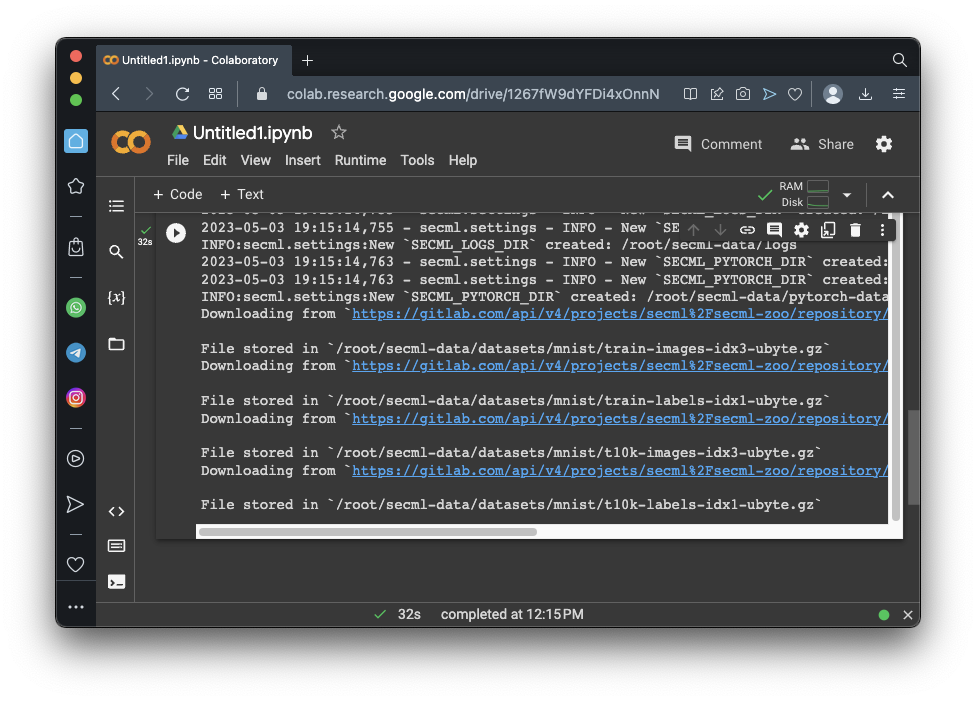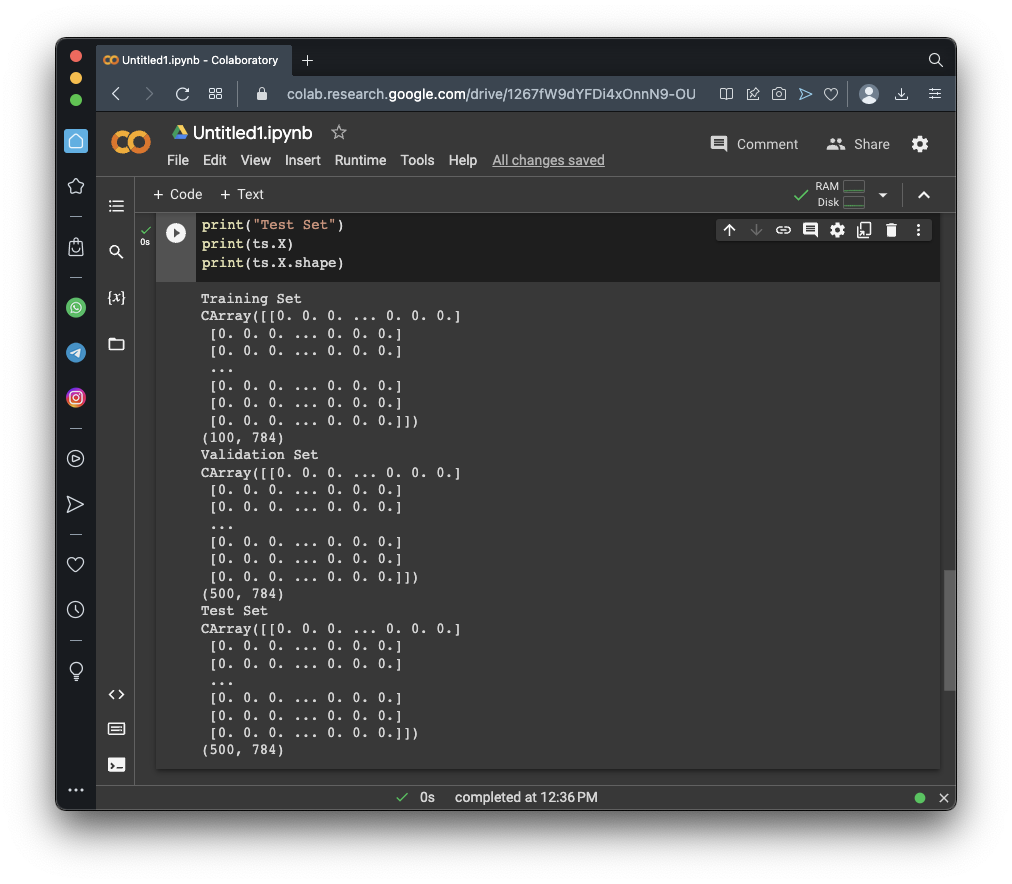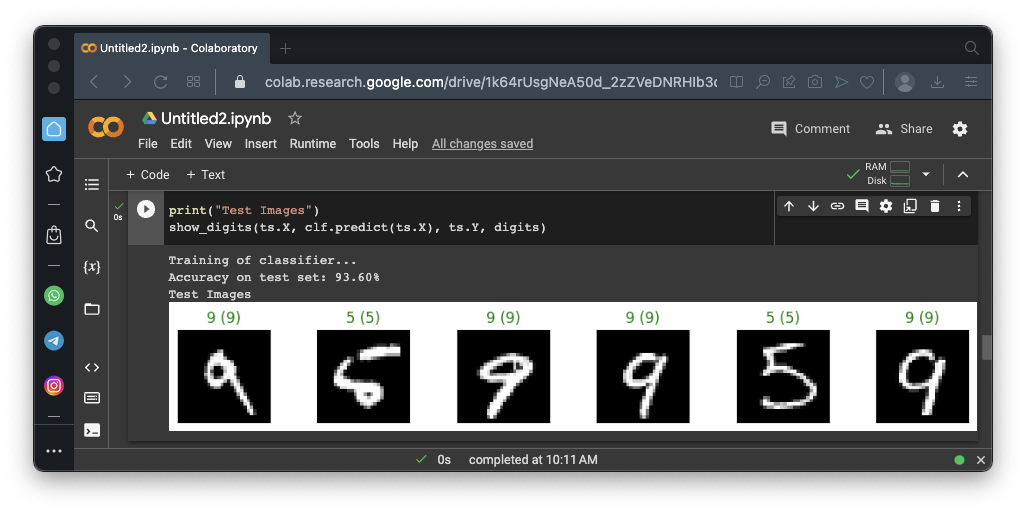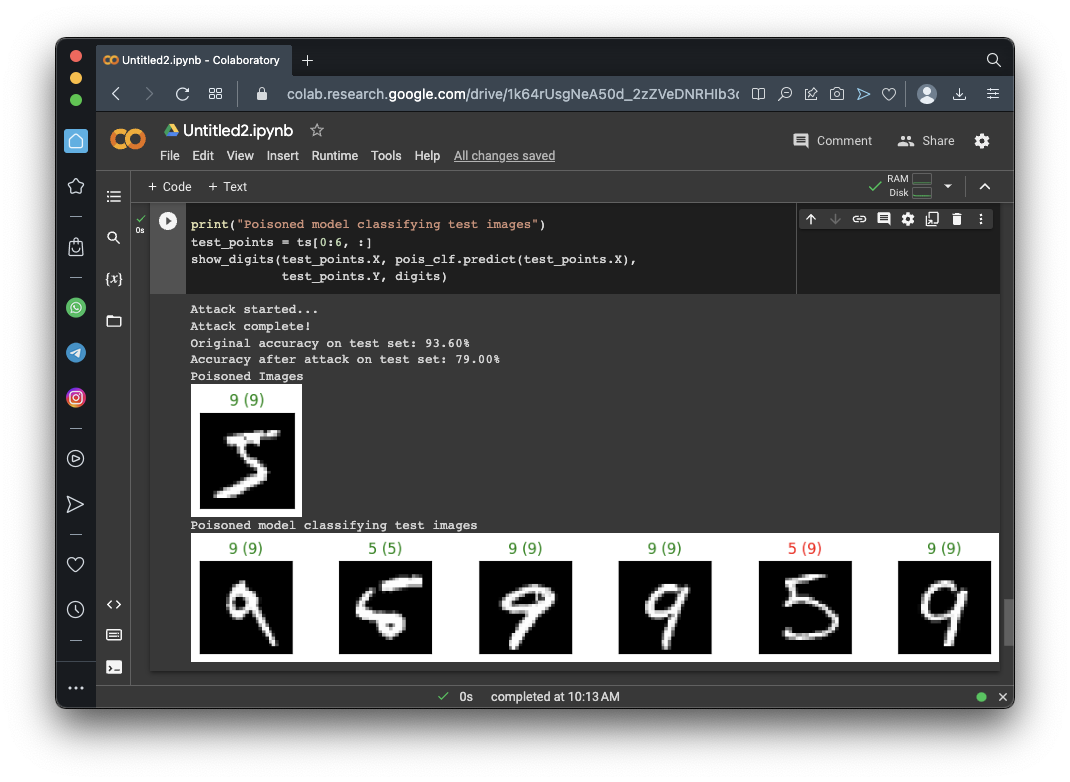# ML 111: Poisoning the MNIST dataset (40 pts extra)

## What You Need

• A Web browser

## Purpose

To practice making simple machine learning code in Python. This project is based on this tutorial:

In a browser, go to

From the menu, click File, "New notebook".

Execute these commands to import the SecML library and download the MNIST dataset, which contains 70,000 small images of handwritten digits.
```!pip install git+https://github.com/pralab/secml import secml from secml.data.loader import CDataLoaderMNIST loader = CDataLoaderMNIST() ```
As shown below, a few pages of messages scroll by, ending with several "File stored" messages.## Selecting Samples to Use

Execute these commands to select a small set of samples, including only the digits 5 and 9.
```random_state = 999 n_tr = 100 # Number of training set samples n_val = 500 # Number of validation set samples n_ts = 500 # Number of test set samples digits = (5, 9) tr_val = loader.load('training', digits=digits, num_samples=n_tr + n_val) ts = loader.load('testing', digits=digits, num_samples=n_ts) # Split in training and validation set tr = tr_val[:n_tr, :] val = tr_val[n_tr:, :] # Normalize the features in `[0, 1]` tr.X /= 255 val.X /= 255 ts.X /= 255 print("Training Set") print(tr.X) print(tr.X.shape) print("Validation Set") print(val.X) print(val.X.shape) print("Test Set") print(ts.X) print(ts.X.shape) ```
As shown below, each set is an array of images, each containing 784 pixels (28x28).## Viewing Samples

Execute these commands to show some examples of the images:
```from secml.figure import CFigure # Only required for visualization in notebooks %matplotlib inline # Let's define a convenience function to easily plot the MNIST dataset def show_digits_1(samples, labels, digs, n_display=6): samples = samples.atleast_2d() n_display = min(n_display, samples.shape) fig = CFigure(width=n_display*2, height=3) for idx in range(n_display): fig.subplot(2, n_display, idx+1) fig.sp.xticks([]) fig.sp.yticks([]) fig.sp.imshow(samples[idx, :].reshape((28, 28)), cmap='gray') fig.sp.title("{}".format(digits[labels[idx].item()])) fig.show() show_digits_1(tr.X, tr.Y, digits) ```
As shown below, the images are handwritten 5's or 9's. The labels above the images show the correct classification.## Training a Classifier

Execute these commands to create and train a model:
```from secml.ml.classifiers import CClassifierSVM # train SVM in the dual space, on a linear kernel, as needed for poisoning clf = CClassifierSVM(C=10, kernel='linear') print("Training of classifier...") clf.fit(tr.X, tr.Y) # Compute predictions on a test set y_pred = clf.predict(ts.X) # Metric to use for performance evaluation from secml.ml.peval.metrics import CMetricAccuracy metric = CMetricAccuracy() # Evaluate the accuracy of the classifier acc = metric.performance_score(y_true=ts.Y, y_pred=y_pred) print("Accuracy on test set: {:.2%}".format(acc)) # Let's define a convenience function to easily plot the MNIST dataset def show_digits(samples, preds, labels, digs, n_display=6): samples = samples.atleast_2d() n_display = min(n_display, samples.shape) fig = CFigure(width=n_display*2, height=3) for idx in range(n_display): fig.subplot(2, n_display, idx+1) fig.sp.xticks([]) fig.sp.yticks([]) fig.sp.imshow(samples[idx, :].reshape((28, 28)), cmap='gray') fig.sp.title("{} ({})".format(digits[labels[idx].item()], digs[preds[idx].item()]), color=("green" if labels[idx].item()==preds[idx].item() else "red")) fig.show() print("Test Images") show_digits(ts.X, clf.predict(ts.X), ts.Y, digits) ```
As shown below, the model is 93.6% accurate, and its predictions (shown in parentheses) are correct for the first six test images.## A Poisoning Attack

Execute these commands to perform an evasion attack--that is, to add malicious training images so that the model will become less accurate. We'll add only one malicious image.
```lb, ub = 0., 1. # Bounds of the attack space. Can be set to `None` for unbounded n_poisoning_points = 1 # Number of poisoning points to generate # Should be chosen depending on the optimization problem solver_params = { 'eta': 0.25, # step size of the gradient descent 'eta_min': 2.0, 'eta_max': None, 'max_iter': 100, 'eps': 1e-6 # tolerance of the stop criterion } from secml.adv.attacks import CAttackPoisoningSVM pois_attack = CAttackPoisoningSVM(classifier=clf, training_data=tr, val=val, lb=lb, ub=ub, solver_params=solver_params, random_seed=random_state) pois_attack.n_points = n_poisoning_points # Run the poisoning attack print("Attack started...") pois_y_pred, _, pois_points_ds, _ = pois_attack.run(ts.X, ts.Y) print("Attack complete!") # Evaluate the accuracy of the original classifier acc = metric.performance_score(y_true=ts.Y, y_pred=clf.predict(ts.X)) # Evaluate the accuracy after the poisoning attack pois_acc = metric.performance_score(y_true=ts.Y, y_pred=pois_y_pred) print("Original accuracy on test set: {:.2%}".format(acc)) print("Accuracy after attack on test set: {:.2%}".format(pois_acc)) # Training of the poisoned classifier for visualization purposes pois_clf = clf.deepcopy() pois_tr = tr.append(pois_points_ds) # Join the training set with the poisoning points pois_clf.fit(pois_tr.X, pois_tr.Y) print("Poisoned Images") show_digits(pois_points_ds.X, pois_clf.predict(pois_points_ds.X), pois_points_ds.Y, digits) print("Poisoned model classifying test images") test_points = ts[0:6, :] show_digits(test_points.X, pois_clf.predict(test_points.X), test_points.Y, digits) ```
As shown below, this single poisoned image caused the accuracy to fall to 79%, and one of the first six test images was classified incorrectly.## Flag ML 111.1: Incorrect Predictions (10 pts)

For the poisoned model above, find the results for the first 25 test images. Find all the incorrect predictions and concatenate their predicted values.

For example, the image above shows that one of the first six test images was incorrectly predicted to be 9. If there were three such images in the first 25 test images, and no other errors, the result would be 999.

That concatenated number is the flag.

## Flag ML 111.2: Poisoning Five Images (10 pts)

Change the attack above to poison five images, as shown below.Construct the flag from the first 25 test images as you did in the previous flag.

## Flag ML 108.3: Optimizing eta (10 pts)

Run the poisoning attack with 30 poisoning images. Try these values of eta: 0.02, 0.05, 0.10, 0.15, 0.25, 0.5, 1.0, and 2.0. Record the accuracy of the poisoned model on the test set for each case.

Use the most effective value of eta--that is, the one that reduces the accuracy the most. Construct the flag by concatenating the incorrect predictions for the first 15 images in the test set.

## Flag ML 111.4: Different Digits (10 pts)

Make these changes:
• Use 200 training samples
• Use 400 validation and test set samples
• Use the digits 1 and 0
• Use 50 poisoning images
• Use eta 0.25
The first four modified images are shown below.Construct the flag by concatenating the incorrect predictions for the first 15 images in the test set.

## Sources

Evasion and Poisoning Attacks on MNIST dataset
secml.optim.optimizers

Posted 5-8-23